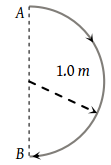You’ve reached the end of your free Videos limit.
#3 -Instantaneous-Velocity--Speed
(Physics) > Motion in A Plane
Related Practice Questions :

In 1.0 s, a particle goes from point A to point B, moving in a semicircle of radius 1.0 m (see figure). The magnitude of the average velocity is(1) 3.14 m/s

(2) 2.0 m/s

(3) 1.0 m/s

(4) Zero

Complete Question Bank + Test Series
Complete Question Bank

Difficulty Level:

The coordinates of a moving particle at any time ‘t’ are given by x = αt3 and y = βt3. The speed of the particle at time ‘t’ is given by

(1) $\sqrt{{\alpha }^{2}+{\beta }^{2}}$

(2) $3\text{\hspace{0.17em}}t\sqrt{{\alpha }^{2}+{\beta }^{2}}$

(3) $3\text{\hspace{0.17em}}{t}^{2}\sqrt{{\alpha }^{2}+{\beta }^{2}}$

(4) ${t}^{2}\sqrt{{\alpha }^{2}+{\beta }^{2}}$

Complete Question Bank + Test Series
Complete Question Bank

Difficulty Level:

Two particles having position vectors $\stackrel{\to }{{r}_{1}}=\left(3\stackrel{^}{i}+5\stackrel{^}{j}\right)$ metres and $\stackrel{\to }{{r}_{2}}=\left(-5\stackrel{^}{i}-3\stackrel{^}{j}\right)$ metres are moving with velocities ${\stackrel{\to }{v}}_{1}=\left(4\stackrel{^}{i}+3\stackrel{^}{j}\right)\text{\hspace{0.17em}}m/s$ and ${\stackrel{\to }{v}}_{2}=\left(\alpha \text{\hspace{0.17em}}\stackrel{^}{i}+7\stackrel{^}{j}\right)$ m/s. If they collide after 2 seconds, the value of ‘α’ is-

(1) 2

(2) 4

(3) 6

(4) 8

Complete Question Bank + Test Series
Complete Question Bank

Difficulty Level:

A particle is moving such that its position coordinates (x, y) are (2m, 3m) at time t=0, (6m, 7m) at time t=2s and (13m, 14m) at time t=5s. Average velocity vector (vav) from t=0 to t=5s is

(a)$\frac{1}{5}$(13$\stackrel{^}{i}$+14$\stackrel{^}{j}$)

(b)$\frac{7}{3}$($\stackrel{^}{i}$+$\stackrel{^}{j}$)

(c)2($\stackrel{^}{i}$+$\stackrel{^}{j}$)

(d)$\frac{11}{5}$($\stackrel{^}{i}$+$\stackrel{^}{j}$)